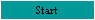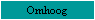## An explanation of the statistics used in the Meta-analysis

By Dr Frans Gieles

 Meta-analysis Sample-level correlation Correlation and effect size Homogeneity Confidence interval The outliers One- and two-tailed tests Weighted means Symptom-level correlation Fisher's Z-transformation

### Meta-analysis

This is an analysis of analyses. The Rind et al. team did not add a new study of a new sample to the existing ones. Meta-analysis is a method to review the data and the results of existing studies. The method makes it possible to compare the data and the results of many other studies and to 'add' the data and the results together, so to speak. By this method, all samples together form a 'new' big sample. This is the strength of a meta-analysis. A statistical rule is: the greater the sample, the more the results can be trusted.

### Correlation and effect size

Correlation is the central concept in the study. Correlation is the association between two or more factors. A factor or a moderator is a force that may have some influence (e.g., intelligence can influence school results). A factor has to be measured by some method. The outcome of the measurement is a variable (e.g., an intelligence quotient).

If a researcher measures the I.Q. of a sample of children, the I.Q. figures will vary among the children. The result of the measurement will show the variability of the sample.

With some methods, one can estimate the variability of the population (e.g. all children of a given age in a given country). Then it's called the population variance.

Analysis of variance or ANOVA, like correlation, measures the association between two or more factors. Put another way, correlation and ANOVA measure how variability in one variable is related to variability in another variable.

The level of correlation is reflected in a correlation coefficient, noted as r, a figure between +1.00 (the longer it rains, the more water in a bin) and -1.00 (the more it rains, the lower the amount of children playing on the streets). The significance (credibility) of this figure depends on the size of the sample, thus on the amount of observations or participants. The more observations for a given value of r, the more significance. Therefore, the number of participants is usually given after the r with the letter n or N.

Note that the size of the association between two variables (i.e., r) is a different concept than statistical significance, which addresses the question of whether or not the two variables are really related to one another. For the meta-analysis, r is used as a measure of effect size.

In a meta-analysis, most of the correlation coefficients are given after a correction in which the size of the sample is included in the calculation. After doing so, a more unbiased r appears: the ru. This figure reflects the best estimate of the level of the correlation within the population.

One useful property of r is that the figure r or ru can be squared. This figure is named the ‘coefficient of determination’ or ‘percentage of variance accounted for’. If some variable V1 predicts 50% of the variability in some variable V2, then the coefficient of determination would be .50 (which corresponds to an r of about .7). Note, that 0.9 x 0.9 = 0.81 and that 0.4 x 0.4 = 0.16. The squared figure ru2 is lower than the ru.

To interpret the effect size, the Rind team calls an r=.50 large, .30 medium, and .10 small. Thus a coefficient of determination of 1% is small, 9% is medium, and 25% is large.

The main factor in the meta-analysis is the experience of CSA. This main factor is compared with many other factors, for example adjustment and many psychological factors. If there appeared to be a high percentage of variance between CSA and, say, adjustment, one supposes that the CSA experience had a (small, medium, or large) effect on the adjustment. If the degree of consent or the gender appears to have effect on the adjustment, than the degree of consent or the gender can be seen as a moderator.

Because the studies gave one effect size for each sample, the number of effect sizes is the same as the number of samples, mentioned in the tables as k.

### Confidence interval

As it has been said: the greater the sample, the more reliable is the correlation. To give a measure for the reliability, usually two figures are given; the one lower and the other higher than the computed correlation coefficient. Between these two figures, the correlation is reliable with a chance of 95% - or a chance of 2.5% that the correlation is lower than the lowest figure and 2.5% that it's higher than the highest figure.

Note that, if the first figure is below zero and the latter above zero, the correlation can be negative as well as positive. If both figures are above zero, we know (with a confidence of 95%) that there is a positive correlation between the given figures, but if one of the figures is zero or negative, we can’t even say with sufficient confidence wether the correlation is negative or positive. This, to cite page 29 of the meta-analysis, "an interval not including zero indicated an effect size estimate was significant."

### One- and two-tailed tests

If the researcher is quite sure that the correlation will be a positive one (as in the example of the wet streets and the rain), he tests only at the positive side of the possible correlation coefficients. This is a one-tailed test. If the researcher is not sure of how two variables are related, or if he wants to know the size of the correlation rather than just its existence or non-existence, he should test at both ends of the possible correlation coefficients: he does a two-tailed test.

### Symptom-level correlation

This is the correlation between several symptoms (for example, depression) and the CSA factor, as it appeared in all samples in which these symptoms are measured. The CSA factor usually has two levels: with or without CSA experience. In other studies, more levels are used, e.g. contact CSA, non-contact CSA, no CSA. The ‘without-group’ is the control group. If, say, 50% of the CSA group had depressive symptoms and also 50% of the control group had depressive symptoms, the effect size of CSA will be zero. If 100% of the CSA group had these symptoms and 0% of the control group, the correlation and the effect size would be 1.00.

### Sample-level correlation

This correlation reflects the overall association between CSA and those types of adjustment measured in the several samples, corrected for the sample size. If a study measured four symptoms in one sample, these four symptom-level effect sizes in the study are averaged into one sample-level effect size in the meta-analysis.

### Homogeneity

A meta-analysis combines the data from several studies about the same subject. Homogeneity measures the differences or similarities between the several studies. If several studies reach nearly the same conclusion, one can combine the data with reasonable confidence. If the studies differ greatly in their outcomes, one should be more cautious about combining the data. The statistical measure of homogeneity between the outcomes of the studies has been given in the tables as H.

This H is calculated by a test, named "Chi-square" that compares the differences between groups of data. The more groups of data, the higher the Chi square will be. The statistical way of saying this is "df (degrees of freedom) = k (number of choices or groups) – 1". To know the significance of the chi-square, one has to look at a table. Usually, the significance is mentioned as an (*) in the tables. An asterisk means that the groups of data were different, a non-significant H suggusts that there was a great deal of homogeneity amongst the several studies. The asterix is explained in the tables as "p < .05 in chi-square test." This means that the cance that such great differences between homologous data would occur is smaller than 5%. To reach homogeneity, the authors removed the most extreme effect sizes, irrespective of wether they were extremely high or extremely low, until homogeneity was reached – if possible. Otherwise, the studies could not be compared with on another with confidence.

### The outliers

Suppose that five studies resulted in the following effect sizes: 0.14, 0.17, 0.23, 0.25 and 0.27. The mean effect size (neglecting the sample size in this example) is 0.21. Now suppose a sixth study resulted in an effect size of 0.70. Then, the mean will be 0.29. The one high effect size will raise the mean and the sixth study would have great influence on the results. It is better to expel this sixth study from the meta-analysis since it seems to be an aberration. These kinds of studied are called "outliers".

Factually, three studies were outliers: two studies with very high positive effect sizes (having many incest cases in the samples) and one with a negative effect size. "Positive" should be read as: "the more CSA, the more problems with adjustment – see page 31 of the meta-analysis.

### Weighted means

If one has a set of effect sizes, one can compute the mean effect size. It is better to include the size of the sample in the computation. Doing so, the larger samples have more influence on the mean than the smaller samples. This mean is called a weighted mean.

### Fisher's-Z transformations

A correlation coefficient r or ru is not an interval measure: i.e. the distance between r = 0.1 to r =0.2 is not the same as the distance from r = 0.8 to r = 0.9. A transformation to Fisher's Z gives each correlation coefficient a figure that better reflects its position in the collection of all coefficients when performing meta-analyses. It makes it possible to use the correlation coefficient and the sample size in a calculation of the weighted mean. This weighted mean can then be transformed back into a correlation coefficient.

BTW, the ru2 or % of variance is an interval measure.

### Standard Deviation or SD

The standard deviation is a figure, mostly between – 2.0 and 2.0, that shows the position of each of the data in the total collection of data. Data with a SD of 0.0 are the mean data. About half of the data have positions between SD – 0.1 and 0.1. Data with positions like – 1.9 or 1.9 are at the extremes of the data collection.

### Multiple regression analysis and (semi) partial correlation

This is a method to compare several ('multiple') factors and to compute the strength of the influence of each of them on another factor. This kind of analysis is better than the 'simple correlation' between only two variables.

Take for example the learning process at school. We can suppose that several factors have influence: the intelligence of the children, the method of teaching, the size of the classes and the personality of the teacher. If you have enough data, you can take the data of the children of the same teacher, the same intelligence and the same class size but with a different method of teaching. Then you 'regress' all factors except one. So you can see if the method of teaching has any influence by computing the correlation between that one factor and the regressed other factors. This correlation is called a partial correlation. With the regression of fewer other factors, it's called a semi partial correlation. By making many of these comparisons, you're doing multiple analysis to compute the strength of each factor. Remember that in the meta-analysis, the factor 'family environment' and 'CSA experience' together had influence on the adjustment, but that 'family environment' appeared to have 10 times more influence than the factor "CSA experience".